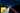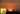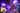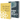# Polynomial Regression and Model Selection

by Robin WieruchAfter learning about , I was curious about using different kinds of hypothesis functions to improve the result of the algorithm itself. So far, the hypothesis function was only a linear straight line. However, your training set may not have a linear correlation and by staying with a linear model it becomes difficult to reduce the cost returned from the cost function.

## Model Selection in a Regression Problem

Often you will get into the topic of machine learning by using linear regression with gradient descent in an univariate training set. One popular training set represents housing prices depending on their sizes in Portland. To keep it simple, for the sake of learning about it, the hypothesis is a straight line through your training set and thus a linear function such as `h(x) => thetaOne * x + thetaZero`.

`Price +      |                                 XXX      |                              XXX      |                    0      XXX     0      |                        XXX    0      |                     XXX      |                  XXX      |               XXX      |     0      XXX      |         XXX      |      XXX       0      |   XXX      |XXX      |      +---------------------------------------+                                           Size`

However, often your features, such as the size and the price of a house, will not have a linear correlation. It's difficult to fit a straight line through the training set in order to predict the prices. The cost function will always return a high value no matter how long you train your algorithm. If you make a wrong decision in the beginning, by selecting an non fitting model type for your regression problem, you may end up with an unsatisfying result.

`Price +      |                        XX |      |       |      |      |                      XX   |      |       |      0      |                    XX     |      0       0      |                  XX |     0      |                XX   |     |      |              XX     |     |      |            XX |     0     0      |     0    XX   |      |     |  XX     |      |  0  |XX       0      |  | XX      |  XX      |XX      +-------------------------------------------------+                                                     Size`

The problem is called under-fitting. In other words, the algorithm has a high bias. Both mean that the prediction doesn't fit the data well. That's where you have to select a different model type to fit your training set in the first place, because the correlation of your x and y values may be nonlinear (e.g. curvilinear).

## Polynomial Regression

Polynomial regression comes into play when your correlation of data is nonlinear and thus a linear model type isn't fitting anymore. Rather than using a straight line, so a linear model to estimate the predictions, it could be for instance a quadratic model or cubic model with a curved line. Polynomial regression is a form of linear regression that allows you to predict a single y variable by decomposing the x variable into a n-th order polynomial. It can have any form of the following function for the hypothesis function.

`h(x) => thetaZero + thetaOne * x + thetaTwo * x^2 + thetaThree * x^3 ..... thetaK * x^k`

As the successive powers of x are added to the equation, the regression line changes its shape. By selecting a fitting model type, you can reduce your costs over time by a significant amount. In the following diagram, the regression line is better fitting than the previously linear regression line.

`Price +      |                                                XX      |                                         XXXXXXX 0      |                                  0    XX 0      |                           0    XXXXXXX      |                           |  XX      |                       XXXXXXX      |                     0X    0      |     0         XXXXXX      |     |       XX|      |  0  |  XXXXX  0      |  |   XX      |  XXXX      |XX      +-------------------------------------------------+                                                     Size`

Polynomial regression can reduce your costs returned by the cost function. It gives your regression line a curvilinear shape and makes it more fitting for your underlying data. By applying a higher order polynomial, you can fit your regression line to your data more precisely. But isn't there any problem with the approach of using more complex polynomials to perfectly fit the regression line?

## Over-fitting in Polynomial Regression

There is one crucial aspect when using polynomial regression. By selecting models for your regression problem, you want to determine which of these models is the most parsimonious. What does a parsimonious model mean? Generally speaking, you need to care more about a parsimony model rather than a best-fitting model. A complex model could over-fit your data. It becomes an over-fitting problem or in other words the algorithm has a high variance. For instance, you might find that a quadratic model fits your trainings set reasonably well. On the other hand, you find out about a very high order polynomial that goes almost perfectly through each of your data points.

`Price +                                            XXX      |                                           X   X      |                               XX         X     X0      |                              X  X0      X0      X      |                           0 X    X     X      |                           |X      X   X      |                   XX      X        XXX      |      XXX         X  0    X0      |     0   X       X    X  X      |    X     X     X      XX      |  0        X   0      | X          XXX      |X      |      +-------------------------------------------------+                                                     Size`

Even though this model fits perfectly, it will be terrible at making future predictions. It fits the data too well, so it's over-fitting. It's about balancing the model's complexity with the model's explanatory power. That's a parsimonious model. It is a model that accomplishes a desired level of explanation or prediction with as few predictor variables as possible. In conclusion, you want to have a best-fitting prediction when using low order polynomials. There is no point in finding the best-fitting regression line that fits all of your data points.

The terminology is used for both linear and logistic regression. In order to address the issue of over-fitting, there are two options:

• Reduce the number of features:
• Manual selection of useful features
• Apply a model selection algorithm
• Regularization (use it, when you have a lot of slightly useful features)
• Keep all the features n, but reduce the magnitude of theta parameters

Hopefully the article helped you to understand the importance of model selection, the polynomial regression and the parsimonious model. I learn about these machine learning topics myself at the moment and try to internalize these learnings by writing about them. If there are any mistakes in the article, please point them out in the comments below so that others and I can learn from it.## Improving Gradient Descent in JavaScript

A recent article gave an introduction to the field of machine learning in JavaScript by predicting housing prices with gradient descent in a univariate regression problem. There are several…## Neural Networks in JavaScript with deeplearn.js

A couple of my recent articles gave an introduction into a subfield of artificial intelligence by implementing foundational machine learning algorithms in JavaScript (e.g. linear regression with…